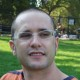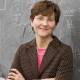# Applied mathematicians vs Theoretical Computer Scientists

[Guest post by Anna Gilbert, who is co-organizing with Piotr Indyk and Dina Katabi a FOCS 2014 workshop on The  Sparse Fourier Transform: Theory and Applications, this Saturday 9am-3:30pm]  After reading Boaz’s post on Updates from the ICM and in particular his discussion of interactions between the TCS and applied math communities, I thought I’d contribute a few observations from my interactions with both, as I consider myself someone who sits right at the intersection. My formal training is in (applied) mathematics and I am currently a faculty member in the Mathematics Department at the University of Michigan. I have spent many years working with TCS people on streaming algorithms and sparse analysis and I worked at AT&T Labs (where the algorithms group was much larger than the “math” group). There are definitely other TCS researchers who are quite adept and interested in collaborations with applied mathematicians, electrical engineers, computational biologists, etc. There are also venues where both communities come together and try to understand what each other is doing. The workshop that Piotr Indyk, Dina Katabi, and I are organizing at FOCS this year is a good example and I encourage anyone interested in learning more about these areas to come. The speakers span a range of areas from TCS, applied math, and electrical engineering! What’s especially fascinating is the juxtaposition of our workshop on the sparse Fourier transform with that of another FOCS workshop that day on Higher-order Fourier Analysis. There are two workshops on Fourier analysis, a topic that is central to applied and computational mathematics, at a conference ostensibly on the Foundations of Computer Science! Here are my observations of both communities (with a large bias towards examples in sparse approximation, compressed sensing, and streaming/sublinear algorithms):

1) Applied mathematicians are not nearly as mathematical as TCS researchers. By which I mean, the careful formal problem statements, the rigorous definitions, the proofs of correctness for an algorithm, the analysis of the use of resources, the definition of resources, etc. are not nearly as developed nor as important to applied mathematicians.

Here are two examples on the importance of clear, formal problem statements and the definition of resources. There a number of different ways to formulate sparse approximation problems, some instantiations are NP-complete and some are not. Some are amenable to convex relaxation and others aren’t. For example, exact sparse approximation of an arbitrary input vector over an arbitrary redundant dictionary is NP-complete but if we draw a dictionary at random and seek a sparse approximation of an arbitrary input vector, this problem is essentially the compressed sensing problem for which we do have efficient algorithms (for suitable distributions on random matrices). Stated this way, it’s clear to TCS what the difference is in the problem formulations but this is not the way many applied mathematicians think about these problems. To the credit of the TCS community, it recognized that randomness is a resource—generating the random matrix in the above example costs something and, should one want to design a compressed sensing hardware device, generating or instantiating that matrix ”in hardware” will cost you resources beyond simple storage. Pseudo-random number generators are a central part of TCS and yet, for many applied mathematicians, they are a small implementation detail easily handled by a function call. Similarly, electrical engineers well-versed in hardware design will use a linear feedback shift register (LFSR) to build such random matrices without making any ”use” of the theory of pseudo-random number generators. The gap between the mathematics of random matrices and the LFSR is precisely where pseudo-random number generators, small space constructions of pseudo-random variables, random variables with limited independence, etc. fit, but forming that bridge and, more importantly, convincing both sides that they need a bridge rather than a simple function call or a simple hardware circuit, is a hard thing to do and not one the TCS community has been successful at. (Perhaps it’s not even something they are aware of.)

2) Many TCS papers, whether they provide algorithms or discuss models of computation that could/should appeal to applied mathematicians, are written or communicated in a way that applied mathematicians can’t/don’t/won’t understand. And, sometimes, the problems that TCS folks address do not resonate with applied mathematicians because they are used to asking questions differently.

My biggest example here is sparse signal recovery as done by TCS versus compressed sensing. For TCS, it is very natural to ask to design both a measurement matrix and a decoding algorithm so that the algorithm returns a good approximation to the sparse representation of the measured signal. For mathematicians, it is much more natural to ask what conditions are sufficient (or, even better, necessary) for the measurement matrix and some existing algorithm (as opposed to one crafted specifically for the problem) to recover the sparse approximation. Applied mathematicians do not, in general, ask questions about how to generate such matrices algorithmically and how to compute with them, unless they are serious about implementing these algorithms and then, typically, these questions are software questions rather than mathematical ones. They are low-level, details, not necessarily abstract questions to be addressed formally. As an even higher level example of a difference in goals, the notion of approximation algorithm is foreign to applied mathematicians—that concept does not appear in numerical analysis. Typically, convergence rates or error analysis for numerical algorithms is expressed as a function of the step-size (for numerical integration, solving differential equations, etc.) or the number of iterations (for any iterative algorithm). It’s standard to seek a bound on the number of iterations one needs to guarantee an error (or relative error) of$\epsilon$ rather than$(1 +\epsilon) \cdot \mathrm{OPT}$. The idea that for the given input, there is an optimal solution and we want our algorithm to return a solution that is close to that optimal is not a standard way of analyzing numerical algorithms. After all, that optimal solution may have terrible error and it’s not easy to determine what the optimal error is.

3) Finally, for many applied mathematicians, computation is a means to an end (e.g., solve the problem, better, faster) as opposed to an equal component of the mathematical problem, one to be studied rigorously for its own sake. And, for a number of TCS researchers, actually making progress on a complicated, real-world problem takes a back seat to the intricate mathematical analysis of the computation. In order for both communities to talk to one another, it helps to understand what matters to each of them.

I think that Michael Mitzenmacher’s response to Boaz’s post is similar to the points in the last point when he says “I think the larger issue is the slow but (over long periods) not really subtle shift of the TCS community away from algorithmic work and practical applications.” Although, I am not sure either model is better. TCS research can be practical, applied math isn’t as useful as we’d like to think, and solving a problem better, faster can be done only after thorough, deep understanding, the type that TCS excels at.

## 3 thoughts on “Applied mathematicians vs Theoretical Computer Scientists”

1.Sanjeev Arora says:

Interesting perspective, Anna.

Some thoughts:

(a) I think you are refering to a small fraction of TCS (and even a small fraction of algorithms research) whose concerns overlap with that of Applied math. Streaming, compressed sensing and related algorithms are the main examples, as you mention.

What about crypto, complexity theory (e.g. you mention approximation; how about showing that certain approximations are not possible?), information/coding, distributed computing, learning theory, graph algorithms, data structures, etc., all of which are parts of TCS? There are no analogous topics in applied math as far as I know.

(b) I think all fields are circumscribed/cocooned to some extent, which prevents them from failing to see the full picture. Usually the founders introduced a bunch of problems and a worldview. 30-40 years later, the problems may change but the worldview remains. And the worldview may be inherently incapable of considering other ways of formalizing and approaching new situations. TCS is no exception, though the broad variety of topics studied in it give it a somewhat larger cocoon.

(c) Implementability of algorithms is indeed something that TCS should re-embrace. To some extent it is liberating to be able to design inefficient algorithms at first and then worry later about making them practical. Not everybody takes the second step nor should they feel forced to (though there are famous practical algorithms that came out of STOC/FOCS work). But more people should do it.

Programming assignments disappeared from TCS courses long ago but are beginning to be reintroduced. I find simple programming assignments quite instructive in my grad algorithms course (geared towards all CS grads, not TCS grads): http://www.cs.princeton.edu/courses/archive/fall13/cos521/
I know of others who are also trying this (eg Tim Roughgarden and Ashish Goel at Stanford). Tools like matlab and scipy make implementations and experimentation much easier.

1.Boaz Barak says:

I took a quick look at the course webpage and assignment and it looks like a fantastic course!

2.annacg says:

Sanjeev,

You’re right, I left out many parts of TCS for several reasons. They don’t have much overlap with applied math (more on that later), I wrote about the parts of both fields I am most familiar with, and Boaz original post was in response to Candes’ talk on an area where, I think, there is quite a bit of overlap.

I think that there are parts of applied math that do make use of some of those other TCS topics you mentioned (e.g., pseudo-random number generators, data structures, perhaps graph algorithms) but those areas are not necessarily elevated to the level of independent, mathematical study. Monte carlo simulations certainly need random number generators! Highly efficient, large scale scientific simulations must leverage good data structure design, computational geometry solutions, nearest neighbor data structures, etc. for efficiency. Again, I don’t know those areas of math all that well but my impression is that the algorithmic contributions are mainly a means to an end.

There is one area that really does seem to have a good meeting of the minds: numerical linear algebra. It’s not an area I know well but only as an observer. After I wrote my post, it occurred to me that it was a good example of fruitful interactions. I hope that the innovative algorithms have an impact on scientific computing too!

Yes, applied math has lived within a cocoon as well for a while. I am just now starting to see students (and faculty) interested in broader areas such as machine learning, bio-informatics (or more discrete parts of mathematical biology), computer vision, image processing of all sorts, and “data stuff.” TCS is far ahead of math in respect to being outward-looking.

I also didn’t mean to give the impression that TCS folks aren’t interested at all in implementations while applied mathematicians are. I’d like to be able to convince the applied math and engineering students I teach that they’d really benefit from an algorithms course, that that sort of course would help them think about their computation more rigorously and thoroughly. I really like all of the innovations in TCS teaching and I am thinking about how to change/update our own numerical analysis classes. Sounds like a combined intro to numerical analysis + algorithms course!